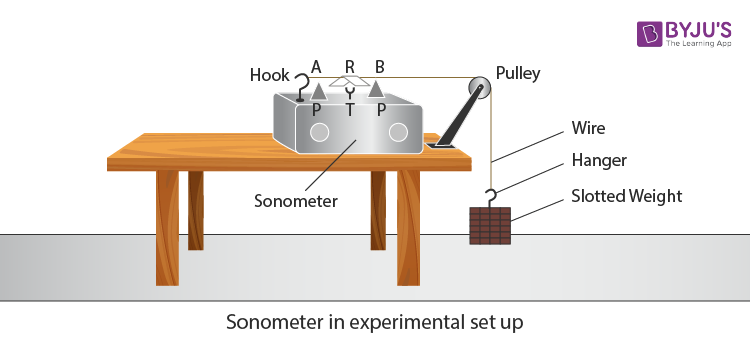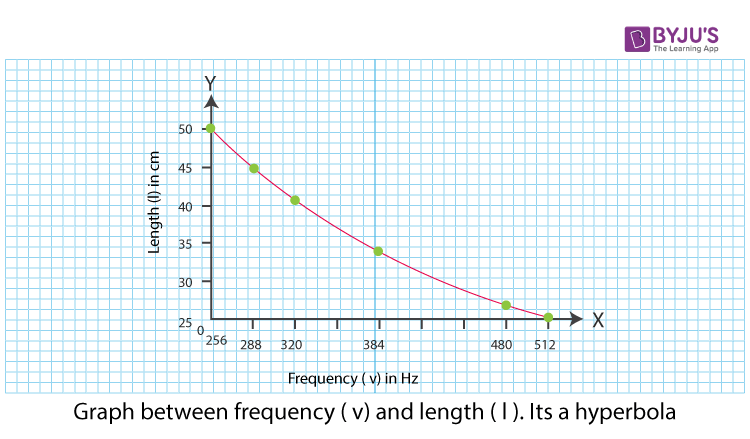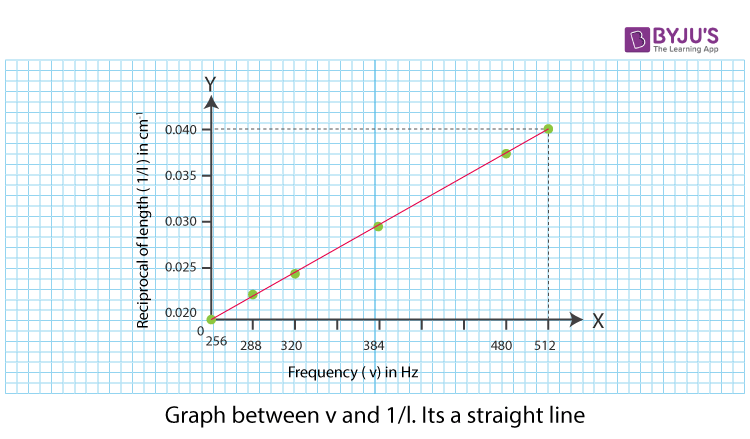# To Study the Relation between Frequency and Length of a Given Wire under Constant Tension Using Sonometer

## Aim

To study the relation between the frequency and the length of a given wire under constant tension using a sonometer.

## Apparatus/Materials Required

• A set of eight tuning forks
• A sonometer
• Seven ½ kg slotted weights
• Paper rider
• Screw gauge
• Metre-scale
• ½ kg hanger

## Diagram## Theory

If the stretched wire vibrates in resonance with the tuning fork with a frequency v, then the string also manifests the same frequency v.

Considering that the string is of length l with diameter D and a density ρ and length l, then the frequency of the string is given by the equation,

$$\begin{array}{l}v=\frac{1}{lD}\sqrt{\frac{T}{\pi \rho }}\end{array}$$

From the above equation, we know that

$$\begin{array}{l}v\propto l\end{array}$$

vl = constant

The graph between v and l will be a hyperbola while the graph between v and 1/l will be a straight line.

The relation between the length (l) and tension (T) from the above equation, is given as follows:

$$\begin{array}{l}\sqrt{\frac{T}{l}}=constant\end{array}$$
$$\begin{array}{l}\sqrt{T}\propto l\end{array}$$
$$\begin{array}{l}T\propto l^2\end{array}$$

A graph between T and l2 will be a straight line

## Procedure

1. Place the sonometer on the table as shown in the figure.
2. Oil the pulley to make it frictionless
3. Put suitable maximum weight to the hanger
4. Move the wooden bridge P outward to include the maximum length of wire AB between them.
5. Pick the tuning fork of the least frequency from the set. Make it vibrate by striking its prong on a rubber pad. Bring the fork close to the ear.
6. Pluck the wire AB from the middle and leave it to vibrate.
7. Listen to the sound produced by both the tuning fork and the wire and judge which has less frequency.
8. Since the long wire may have less frequency move the bridges inward and check the frequencies again.
9. Go on decreasing the length of the wire till the frequency of the wire becomes equal to the frequency of the tuning fork.
10. Place an inverted V-shaped paper rider in the middle of the wire AB. Vibrate the tuning fork and touch the lower end of its handle with a sonometer board. The wire AB vibrates due to resonance and the paper rider falls.
11. Note the length of the wire AB between the edges of two bridges and record it in the ‘length decreasing’ column.
12. Bring the bridges closer and adjust the length of the wire by increasing little by little till the rider falls.
13. Note the length of the wire and record it in ‘length increasing column.’
14. Take the rest of the five tuning forks, and one by one, in the order of increasing frequency repeat steps 5 to 13.
15. Record the observations as given below.

## Observations

Constant tension on the wire, T = _____ kg.

 Serial No of observations Frequency of tuning fork used v (Hz) Resonant length of wire 1/l cm–1 Length increasing (l1) Length decreasing(l2) $$\begin{array}{l}\frac{l_1+l_2}{2}\end{array}$$

Table for frequency and length

## Calculations

1. Find the mean length l.
2. Determine 1/l
3. Plot a graph between v and l, with v along the x-axis and l along the y-axis.1. Now, plot a graph between v and 1/l, with v along the x-axis and 1/l along the y-axis. The graph is a straight line as shown below.## Result

From the graph, it can be concluded that vl = constant and

$$\begin{array}{l}v\propto \frac{1}{l}\end{array}$$
.

## Viva Voce

1.What is the principle involved in sonometer?

Answer: Resonance is the principle involved in the sonometer.

2. Which type of waves are produced in the sonometer experiment?

Answer: Transverse waves are produced in the sonometer experiment.

3. What is the relationship between frequency and the length of a given wire under constant tension?

Answer: The relationship between the frequency and the length of a given wire under constant tension is given by the equation

$$\begin{array}{l}v=\frac{1}{lD}\sqrt{\frac{T}{\pi \rho }}\end{array}$$

## Frequently Asked Questions – FAQs

Q1

### What is the principle involved in a sonometer?

Resonance is the principle involved in the sonometer.
Q2

### Which type of waves are produced in the sonometer experiment?

Transverse waves are produced in the sonometer experiment.
Q3

### What is the relationship between frequency and the length of a given wire under constant tension?

The relationship between the frequency and the length of a given wire under constant tension is given by the equation.